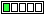All about flooble | fun stuff | Get a free chatterbox | Free JavaScript | Avatarsperplexus dot infoTrigonometry Nest 2 (Posted on 2005-11-07)In Trigonometry Nest, the functions sin(cos(x)) and cos(sin(x)) were introduced, and it was proven that one of these functions is always greater than the other.

Taking this concept to infinity, which of these functions is greater: C(x)=cos(sin(cos(......(sin(x))))...))), or S(x)=sin(cos(sin(......(cos(x))))...)))?

How does the ratio C(x)/S(x) depend on x?

 See The Solution Submitted by Larry Rating: 3.0000 (2 votes)Comments: ( Back to comment list | You must be logged in to post comments.)Excel spoiler| Comment 1 of 7
As long as nobody was commenting, I thought I'd try it in excel, just to see whether it really converged.

In fact, it seems to converge pretty quickly.

I have C(x) approximates .768169 for all x
S(x) approximates .694820 for all x

C(x) is greater

 Posted by Steve Herman on 2005-11-07 10:22:32Please log in:

 Search: Search body:
Forums (0)